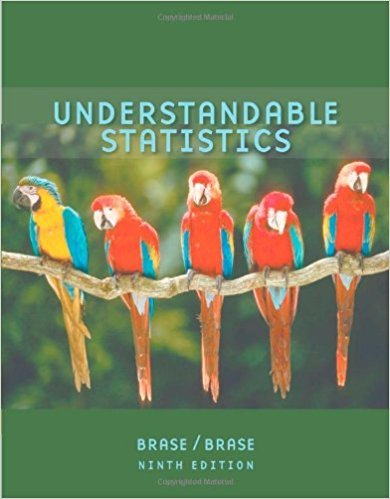×
×

# Solutions for Chapter 12: NONPARAMETRIC STATISTICS## Full solutions for Understandable Statistics | 9th Edition

ISBN: 9780618949922Solutions for Chapter 12: NONPARAMETRIC STATISTICS

Solutions for Chapter 12
4 5 0 242 Reviews
10
4
##### ISBN: 9780618949922

Since 10 problems in chapter 12: NONPARAMETRIC STATISTICS have been answered, more than 35901 students have viewed full step-by-step solutions from this chapter. This textbook survival guide was created for the textbook: Understandable Statistics, edition: 9. Chapter 12: NONPARAMETRIC STATISTICS includes 10 full step-by-step solutions. This expansive textbook survival guide covers the following chapters and their solutions. Understandable Statistics was written by and is associated to the ISBN: 9780618949922.

Key Statistics Terms and definitions covered in this textbook
• Alternative hypothesis

In statistical hypothesis testing, this is a hypothesis other than the one that is being tested. The alternative hypothesis contains feasible conditions, whereas the null hypothesis speciies conditions that are under test

• Arithmetic mean

The arithmetic mean of a set of numbers x1 , x2 ,…, xn is their sum divided by the number of observations, or ( / )1 1 n xi t n ? = . The arithmetic mean is usually denoted by x , and is often called the average

• Bivariate normal distribution

The joint distribution of two normal random variables

• C chart

An attribute control chart that plots the total number of defects per unit in a subgroup. Similar to a defects-per-unit or U chart.

• Causal variable

When y fx = ( ) and y is considered to be caused by x, x is sometimes called a causal variable

• Comparative experiment

An experiment in which the treatments (experimental conditions) that are to be studied are included in the experiment. The data from the experiment are used to evaluate the treatments.

• Components of variance

The individual components of the total variance that are attributable to speciic sources. This usually refers to the individual variance components arising from a random or mixed model analysis of variance.

• Confounding

When a factorial experiment is run in blocks and the blocks are too small to contain a complete replicate of the experiment, one can run a fraction of the replicate in each block, but this results in losing information on some effects. These effects are linked with or confounded with the blocks. In general, when two factors are varied such that their individual effects cannot be determined separately, their effects are said to be confounded.

• Control chart

A graphical display used to monitor a process. It usually consists of a horizontal center line corresponding to the in-control value of the parameter that is being monitored and lower and upper control limits. The control limits are determined by statistical criteria and are not arbitrary, nor are they related to speciication limits. If sample points fall within the control limits, the process is said to be in-control, or free from assignable causes. Points beyond the control limits indicate an out-of-control process; that is, assignable causes are likely present. This signals the need to ind and remove the assignable causes.

• Cook’s distance

In regression, Cook’s distance is a measure of the inluence of each individual observation on the estimates of the regression model parameters. It expresses the distance that the vector of model parameter estimates with the ith observation removed lies from the vector of model parameter estimates based on all observations. Large values of Cook’s distance indicate that the observation is inluential.

• Critical region

In hypothesis testing, this is the portion of the sample space of a test statistic that will lead to rejection of the null hypothesis.

• Cumulative distribution function

For a random variable X, the function of X deined as PX x ( ) ? that is used to specify the probability distribution.

• Defect concentration diagram

A quality tool that graphically shows the location of defects on a part or in a process.

• Deming

W. Edwards Deming (1900–1993) was a leader in the use of statistical quality control.

• Design matrix

A matrix that provides the tests that are to be conducted in an experiment.

• Distribution function

Another name for a cumulative distribution function.

• Error variance

The variance of an error term or component in a model.

• Extra sum of squares method

A method used in regression analysis to conduct a hypothesis test for the additional contribution of one or more variables to a model.

• Fractional factorial experiment

A type of factorial experiment in which not all possible treatment combinations are run. This is usually done to reduce the size of an experiment with several factors.

• Generator

Effects in a fractional factorial experiment that are used to construct the experimental tests used in the experiment. The generators also deine the aliases.

×